# Draw Color Filled Shapes in Turtle – Python

Prerequisite: Python Turtle Basics

turtle is an inbuilt module in python. It provides drawing using a screen (cardboard) and turtle (pen). To draw something on the screen, we need to move the turtle. To move turtle, there are some functions i.e `forward()`, `backward()`, etc.

To fill the colors in the shapes drawn by turtle, turtle provides three functions –

fillcolor(): This helps to choose the color for filling the shape. It takes the input parameter as the color name or hex value of the color and fills the upcoming closed geographical objects with the chosen color. Color names are basic color names i.e. red, blue, green, orange.
The hex value of color is a string(starting with ‘#’) of hexadecimal numbers i.e. #RRGGBB. R, G, and B are the hexadecimal numbers (0, 1, 2, 3, 4, 5, 6, 7, 8, 9, A, B, C, D, E, F).

begin_fill(): This function tells turtle that all upcoming closed graphical objects needed to be filled by the chosen color.

end_fill(): this function tells turtle to stop the filling upcoming closed graphical objects.

## Drawing Color Filled Square:

 `# draw color-filled square in turtle ` ` `  `import` `turtle ` ` `  `# creating turtle pen ` `t ``=` `turtle.Turtle() ` ` `  `# taking input for the side of the square ` `s ``=` `int``(``input``(``"Enter the length of the side of the square: "``)) ` ` `  `# taking the input for the color ` `col ``=` `input``(``"Enter the color name or hex value of color(# RRGGBB): "``) ` ` `  `# set the fillcolor ` `t.fillcolor(col) ` ` `  `# start the filling color ` `t.begin_fill() ` ` `  `# drawing the square of side s ` `for` `_ ``in` `range``(``4``): ` `  ``t.forward(s) ` `  ``t.right(``90``) ` ` `  `# ending the filling of the color ` `t.end_fill() `

Input :

```200
green```

Output :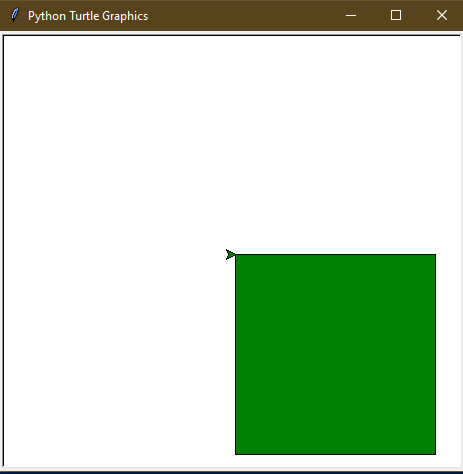## Drawing Color Filled Triangle:

 `# draw color filled triangle in turtle ` ` `  `import` `turtle ` ` `  `# creating turtle pen ` `t ``=` `turtle.Turtle() ` ` `  `# taking input for the side of the triangle ` `s ``=` `int``(``input``(``"Enter the length of the side of the triangle: "``)) ` ` `  `# taking the input for the color ` `col ``=` `input``(``"Enter the color name or hex value of color(# RRGGBB): "``) ` ` `  `# set the fillcolor ` `t.fillcolor(col) ` ` `  `# start the filling color ` `t.begin_fill() ` ` `  `# drawing the triangle of side s ` `for` `_ ``in` `range``(``3``): ` `  ``t.forward(s) ` `  ``t.right(``-``120``) ` ` `  `# ending the filling of the color ` `t.end_fill() `

Input :

```200
red```

Output :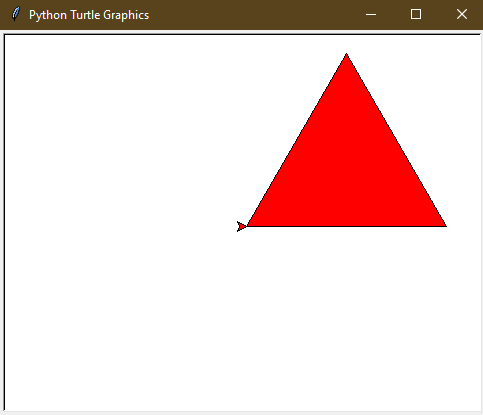## Drawing Color Filled Hexagon:

 `# draw color-filled hexagon in turtle ` ` `  `import` `turtle ` ` `  `# creating turtle pen ` `t ``=` `turtle.Turtle() ` ` `  `# taking input for the side of the hexagon ` `s ``=` `int``(``input``(``"Enter the length of the side of the hexagon: "``)) ` ` `  `# taking the input for the color ` `col ``=` `input``(``"Enter the color name or hex value of color(# RRGGBB): "``) ` ` `  `# set the fillcolor ` `t.fillcolor(col) ` ` `  `# start the filling color ` `t.begin_fill() ` ` `  `# drawing the hexagon of side s ` `for` `_ ``in` `range``(``6``): ` `  ``t.forward(s) ` `  ``t.right(``-``60``) ` ` `  `# ending the filling of the color ` `t.end_fill() `

Input :

```100
#113300```

Output :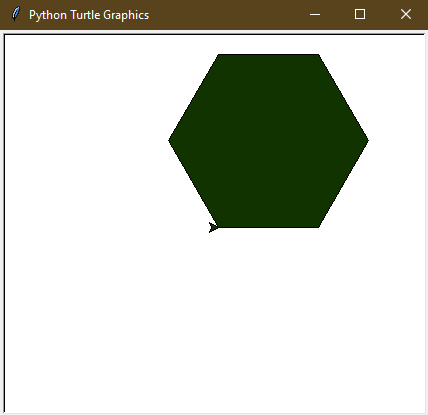## Drawing Color Filled Star:

 `# draw color filled star in turtle ` ` `  `import` `turtle ` ` `  `# creating turtle pen ` `t ``=` `turtle.Turtle() ` ` `  `# taking input for the side of the star ` `s ``=` `int``(``input``(``"Enter the length of the side of the star: "``)) ` ` `  `# taking the input for the color ` `col ``=` `input``(``"Enter the color name or hex value of color(# RRGGBB): "``) ` ` `  `# set the fillcolor ` `t.fillcolor(col) ` ` `  `# start the filling color ` `t.begin_fill() ` ` `  `# drawing the star of side s ` `for` `_ ``in` `range``(``5``): ` `  ``t.forward(s) ` `  ``t.right(``144``) ` ` `  `# ending the filling of color ` `t.end_fill() `

Input :

```200
#551122```

Output :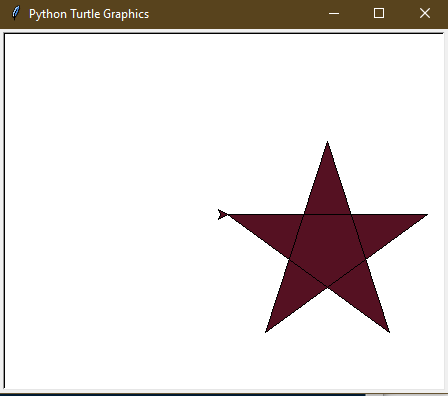## Drawing Color Filled Circle:

 `# draw color filled circle in turtle ` ` `  `import` `turtle ` ` `  `# creating turtle pen ` `t ``=` `turtle.Turtle() ` ` `  `# taking input for the radius of the circle ` `r ``=` `int``(``input``(``"Enter the radius of the circle: "``)) ` ` `  `# taking the input for the color ` `col ``=` `input``(``"Enter the color name or hex value of color(# RRGGBB): "``) ` ` `  `# set the fillcolor ` `t.fillcolor(col) ` ` `  `# start the filling color ` `t.begin_fill() ` ` `  `# drawing the circle of radius r ` `t.circle(r) ` ` `  `# ending the filling of the color ` `t.end_fill() `

Input :

```100
blue```

Output :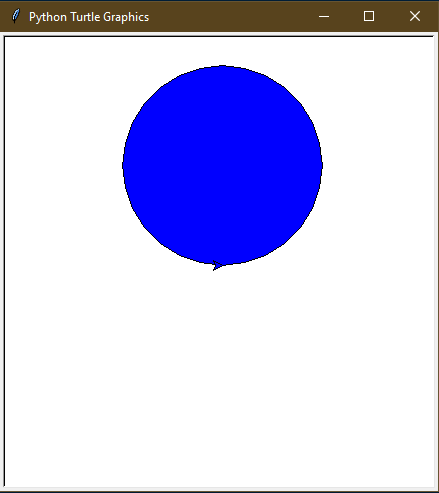Attention geek! Strengthen your foundations with the Python Programming Foundation Course and learn the basics.

To begin with, your interview preparations Enhance your Data Structures concepts with the Python DS Course.

My Personal Notes arrow_drop_upCheck out this Author's contributed articles.

If you like GeeksforGeeks and would like to contribute, you can also write an article using contribute.geeksforgeeks.org or mail your article to contribute@geeksforgeeks.org. See your article appearing on the GeeksforGeeks main page and help other Geeks.

Please Improve this article if you find anything incorrect by clicking on the "Improve Article" button below.

Article Tags :

1

Please write to us at contribute@geeksforgeeks.org to report any issue with the above content.